# Phase Response and Receive Delay

By Charlie Hughes

## Some time-of-flight compensation guidelines for proper viewing of the loudspeaker’s phase response.

At the recent EQ07 workshop, and on the SAC email discussion group afterwords, there were some questions about the “correct” phase response when measuring a loudspeaker or some other band pass device. I thought it might be informative to review setting the receive delay within a measurement system so that the displayed phase response is the “correct” one.

I have correct in quotes above because for a given magnitude response there are an infinite number of equally valid phase responses. This is due in part to what is called propagation delay. This is simply the time of flight of a signal through the air or any other medium. It can also be caused by the latency of a DSP unit. An increase in propagation delay, or any frequency independent delay for that matter, increases the phase shift of a signal at the receiver location compared to when it originated. This is shown in Figure 1. The same device was measured three different times with a different propagation delay for each measurement. This could have been due to different microphone location or different DSP latency.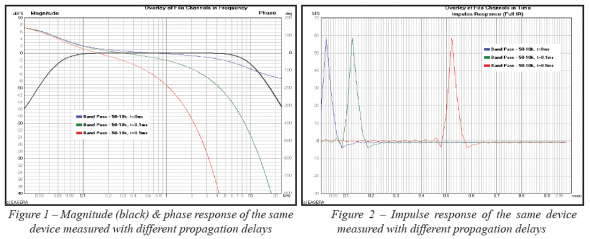In this case a different signal delay was applied to illustrate the point. In each case the magnitude response is the same but the phase response is different. The reason for this can be understood by looking Figure 2. This shows the impulse response, in the time domain, of these same measurements. The progressively later arrival of each impulse (more propagation delay) causes more phase shift.

To see the inherent phase response of a DUT we would like to be able to remove all of the propagation delay (red and green traces in Figure 1 & Figure 2) so that we are left with only the phase shift caused by the DUT itself (blue trace in Figure 1 & Figure 2). But exactly how much time compensation (receive delay) should be used? If we don’t remove all of the propagation delay the phase response will continue to decrease, particularly in the high frequency region. If we remove too much propagation delay the phase response will increase, again predominantly in the high frequency region. Both of these conditions are shown in Figure 3. This latter condition indicates that energy came out of the DUT before it went into it. This is referred to as an a causal response meaning that the output was not caused by the input. This is shown in Figure 4 as the impulse arriving before time t=0. This can’t happen in real time but can be made to appear as if it did with different analyzer settings or post processing.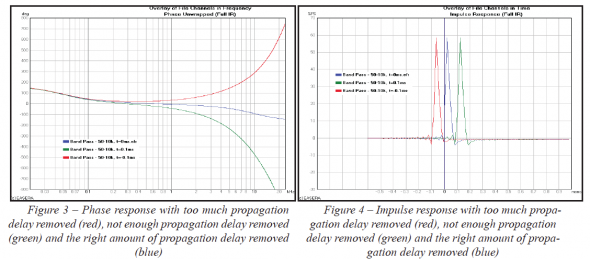A loudspeaker can be modeled as a band pass filter. At low frequency the magnitude response will be very low in level and increasing with increasing frequency until it reaches its pass band level and flattens out. At a higher frequency the magnitude response will begin to decrease with increasing frequency. This is shown in Figure 5. The phase response for this DUT is also shown.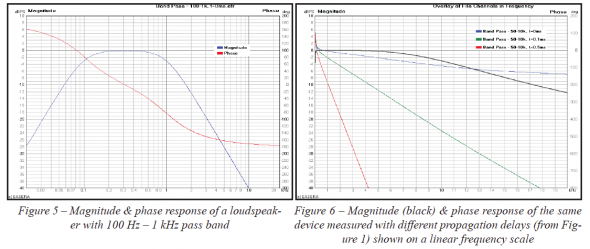In this example, a 1 kHz corner frequency is selected as the high frequency limit to illustrate a concept detailed in a paper by Richard Heyser1. This two page paper is highly recommended reading. Its brevity greatly belies its substance.

Heyser stated that “If the value of time delay2 in the measuring medium is to be subtracted in the measurement process, as can be done for impulse, cross spectrum and TDS measurements, then the proper value of time delay has been subtracted, and the true acoustic position for the phase measurement has been obtained, when the plot of phase angle versus frequency approaches a flat horizontal line for frequencies well outside the pass band of the DUT.” In other words we have removed the correct propagation delay when the phase response in the frequency region well above the cutoff frequency of the DUT approaches a horizontal line. This is exactly what is beginning to occur in Figure 5 at approximately 8 kHz and higher. The phase response is asymptotically approaching a horizontal line.

Notice also that in the higher frequency region of the pass band and just beyond (500 – 2,000 Hz) the phase response is not flat. It is erroneous, albeit possible, to set a receive delay to yield a nearly horizontal phase response in this frequency region. This is a very common error and one that was formerly perpetrated by the author with some regularity.

Now that we know what to look for in the phase response, how do we achieve it? We can look at Figure 2 or Figure 4 and conclude that the correct receive delay to apply is some time between the initial perturbation of the impulse response from a magnitude of 0 (or out of the noise floor) and its maximum value. We could iteratively try each incremental data point (sample) of the IR and look at the phase to see if we had it right. This may become quite time consuming, however. Alternatively, we can use group delay to help us determine the correct receive delay.

Remember from previous SAC Newsletter articles (Vol. 34, No. 4 and Vol. 35, No. 2) that group delay is the negative rate of change of phase with respect to frequency.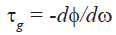In this equation the Greek letter omega (ω) denotes angular frequency which is simple 2π times frequency. The Greek letters tau (τ) and phi (φ) denote group delay and phase, respectively. This is just the mathematical way of saying that the negative slope of the phase response gives us the group delay response. This means that if the slope of the phase response is constant, i.e. a straight line, over a range of frequencies then the group delay has a constant value over that same range of frequencies.

In real time the phase response always slopes down with increasing frequency. This means the slope is negative. If this actual slope is used as the group delay it implies that group delay is negative; that is the signal arrives at the mic/listener at some negative time (prior to when it left the loudspeaker). Since this can’t happen in real time we negate the slope (multiply it by -1) of the phase response to get group delay. A negative slope times -1 will always yield a positive group delay (the signal arrives at the mic/listener at some time after it left the loudspeaker).

To see the constant slope of the phase response we must view it on a linear frequency scale. When viewed on the more common logarithmic frequency scale the phase response appears to curve down more in the high frequency region. This is simply a result of viewing phase on a log frequency scale. The phase response curves of Figure 1 are shown on a linear frequency scale in Figure 6. Here we see that progressively greater propagation delay results in a straight line (in the high frequency region) with progressively greater downward slope (compare Figure 6 with Figure 2).

The group delay for these phase responses is shown in Figure 7. The group delay in the very high frequency region of each of these curves approaches the actual propagation delay for each measurement.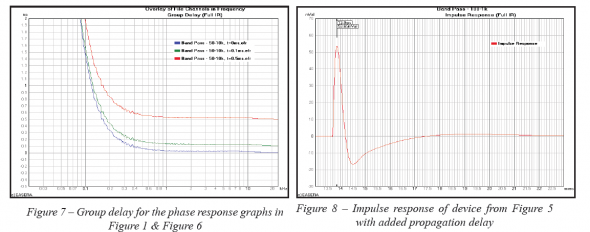Let’s return to our example in Figure 5 for a moment. This has a much lower cutoff frequency (high frequency limit) than the example in Figure 1. Since it has less high frequency output and more phase shift as seen in the frequency domain, the impulse response (or the ETC) in the time domain will have a longer rise time. This makes it more difficult to determine its true propagation delay from these time domain graphs. The cursor in Figure 8 shows the peak arrival at 13.9 ms. However, the cursor at 8 kHz on the group delay response shown in Figure 9 indicates the propagation delay to be 13.71 ms. This is the true propagation delay for this measurement.

If we had used 13.9 ms as the receive delay we would have removed 190 us (microseconds) more than the actual propagation time. This would have resulted in the phase response turning up and increasing with increasing frequency. This is shown in Figure 10 along with the phase response using a 13.71 ms receive delay. Comparing this to Figure 5 should be convincing.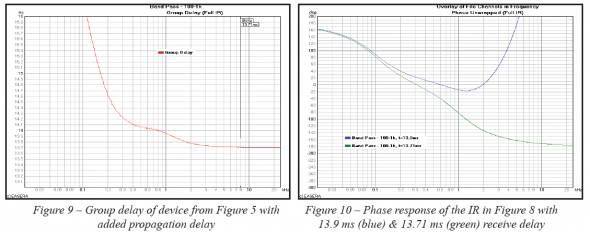We have seen that the proper receive delay to be used for removing propagation delay is not the time corresponding to the maximum value (peak) of the IR (or ETC) but typically a bit earlier. We have also seen that this time can be located fairly accurately by looking at the group delay. Using these techniques we have also seen that the phase response of a system with a band pass response, typically of loudspeakers, does not have flat phase response in the high frequency region of its pass band. It is only at higher frequencies, well above its pass band, that the inherent phase response of a device begins to approach a flat, horizontal line. ch

1 “Determining the Acoustic Position for Proper Phase Response of Transducers”, JAES, vol. 32 no. 1/2, pp. 23-25 (Jan/Feb 1984); also in Time Delay Spectrometry – An Anthology of the Works of Richard C. Heyser, pp. 182-183

2 The term time delay was commonly used in the past. Today we more accurately refer to this as signal delay. As Don Davis pointed out many years ago, “One may delay a signal but one may not delay time.”

Tags: